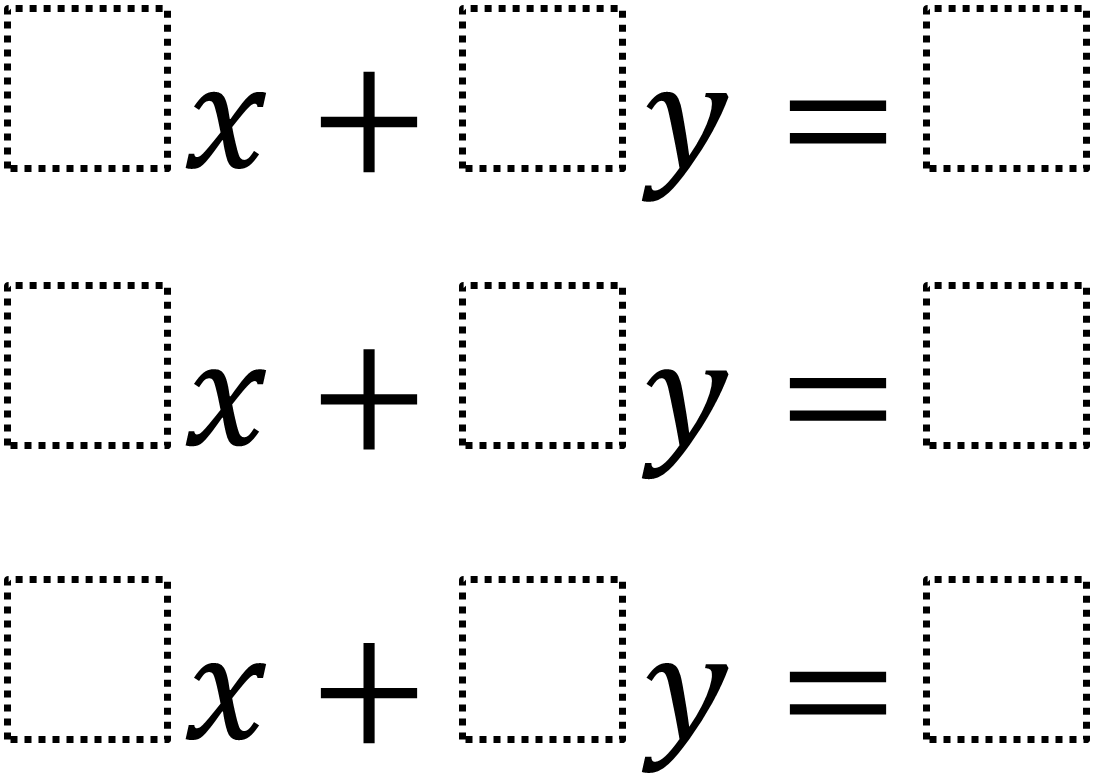# Parallel Lines and Perpendicular Transversals

Directions: Using the digits 1 to 9 at most one time each, fill in the boxes so that 2 of the lines are parallel and the third line is a transversal that is as close to perpendicular to the parallel lines as possible.### Hint

What do we know about the slopes of parallel lines and transversals?

Since the slope of a perpendicular line should be the negative reciprocal of the other line, and since we can’t make it negative, then the slope that is closest to zero should be the closest to perpendicular.  Accordingly, the best answer so far is 6x + 3y = 7, 4x + 2y = 8, x + 9y = 5

Source: Shelli Foust and Robert Kaplinsky

## Similar Triangles 2

Directions: Using the digits 0 to 9 at most one time each, create two similar …

1.•